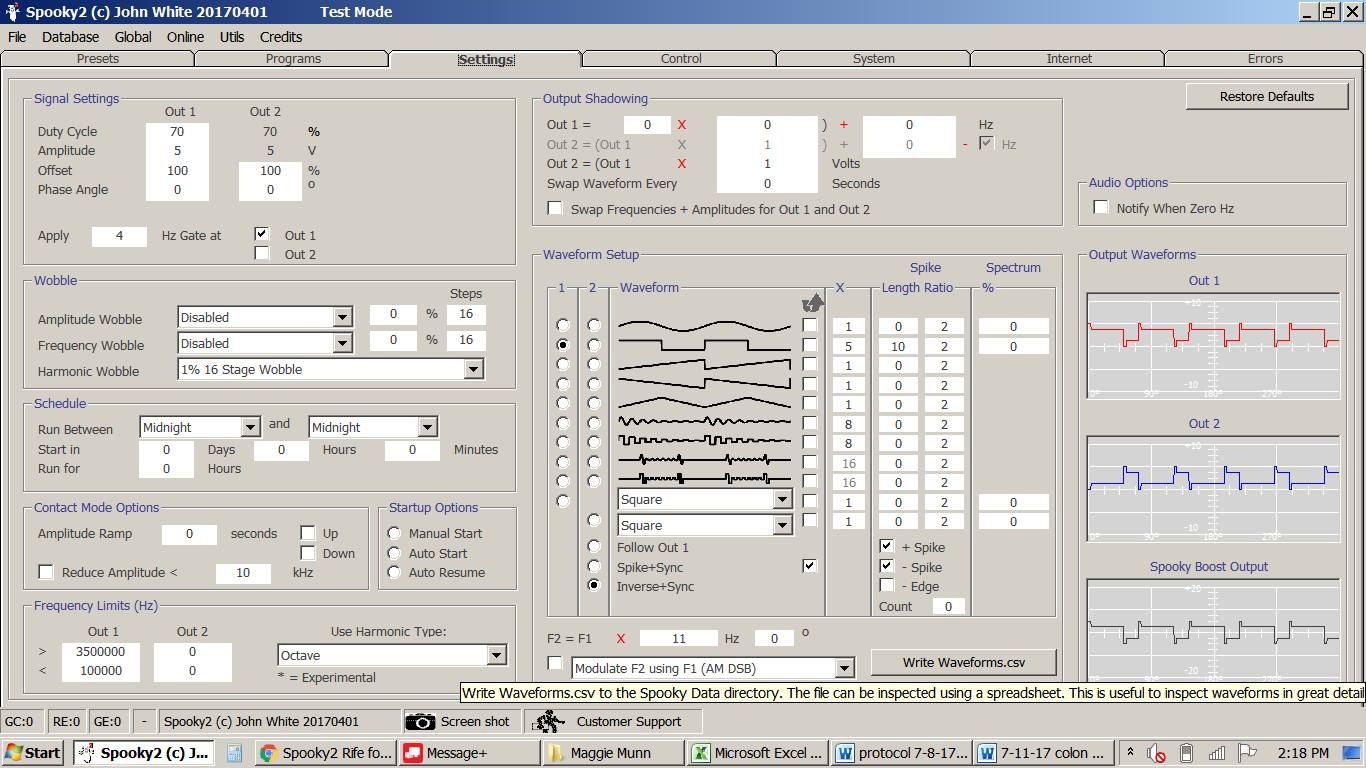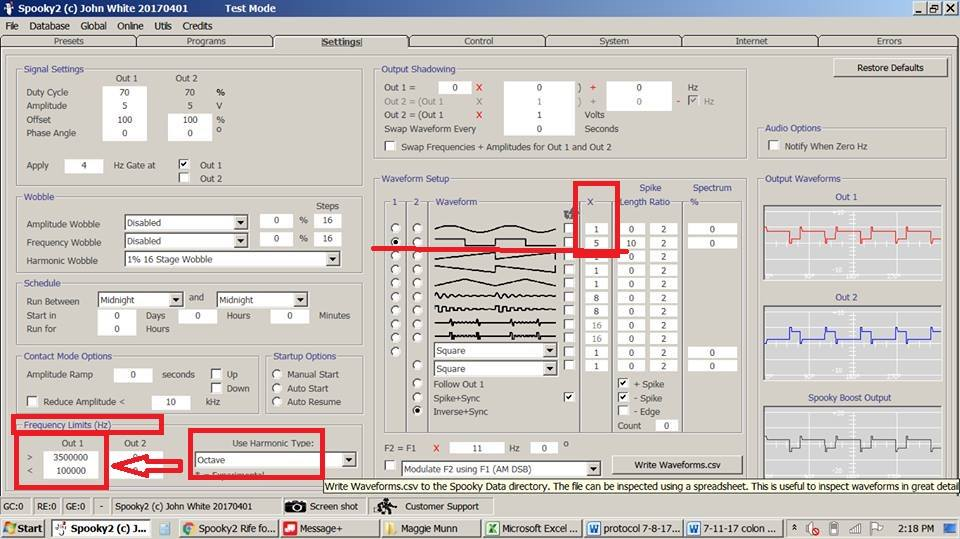If I want run on Spooky2 Central with plasma and hand cylinders,is it right that the numbers I put in my program?Yes. The frequencies are probably correct. If you look in the lower left of your screenshot, you see the Frequency Limits box. This box tells the software to make all output frequencies between 100,000 and 3,500,000 or 100K to 3.5MHz. It does this by multiplying the frequency by 8 (Octave) until it is larger than 100K.

See the numbers lower left in the box, this shows the frequency range that the output frequencies MUST be between. It also shows the method to calculate the specific frequencies, as Octave.

Another possible confusion factor here is that the X multiplier is 5, which means that all frequencies will be divided by five, and the waveform will have 5 cycles within it - to make it easier for the generator to make the higher frequencies.

So, I think the way you would have to verify the frequencies to the generator is:
program frequency divided by 5, then multiply times 8 until the result is higher than 100,000Hz - and it should match what is sent to the generator.For more details, please check the link: